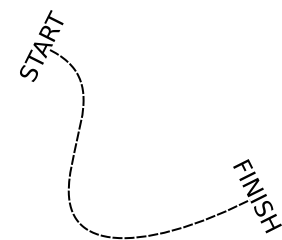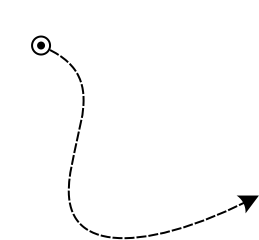## 8.1 Markers

The function path-transform-as-marker rotates, scales and translates a path to one of the ends of another path. This allows to use any path as marker. The example below use 2 strings.

```(zpb-ttf:with-font-loader (font "vera.ttf")
(let ((p1 (create-path :open-polyline))
(p2 (make-string-path font "START"
:size 24 :halign :center :valign :bottom))
(p3 (make-string-path font "FINISH"
:size 24 :halign :center :valign :bottom)))
(path-reset p1 (make-point 50 50))
(path-extend p1 (make-bezier-curve (list (make-point 150 100)
(make-point 0 200)
(make-point 50 300)))
(make-point 250 200))
(show-path (stroke-path (dash-path p1 #(10 2)) 2.0)
(path-transform-as-marker p2 p1 :begin)
(path-transform-as-marker p3 p1 :end))))
```

produces:With two paths defined as follow:

```(defun make-marker1 ()
(list (make-circle-path 0 5 5)
(path-reversed (make-circle-path 0 5 4))
(make-circle-path 0 5 2)))

(defun make-marker2 ()
(let ((path (create-path :polygon)))
(path-extend path (make-arc 10 10) (make-point 5 -5))
(path-extend path (make-straight-line) (make-point 0 5))
(path-extend path (make-straight-line) (make-point -5 -5))
path))
```

used in place of p2 and p3 in the previous examples, produces:Generated by CL-Crock on 2010-09-25T15:20:59Z